# Solution assignment 03 Addition and subtraction formulas

### Assignment 3

Solve for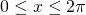: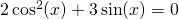### Solution

We substitute in the equation: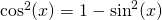and get: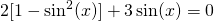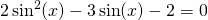This is a quadratic equation in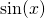. We use the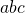-formula: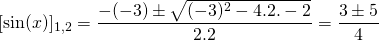Thus the solutions are: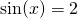of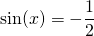The first equation cannot give solutions becausecannot be greater than.
For the second equation we have: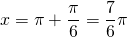or: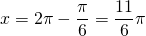0